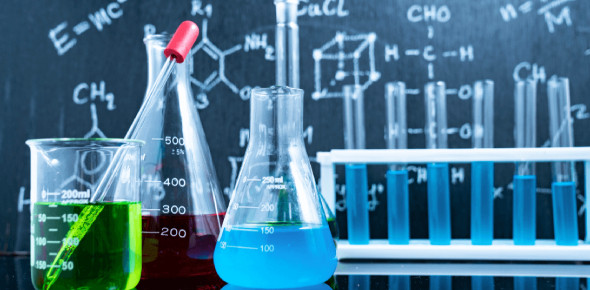# Toughest Chemistry Exam Quiz: Trivia!

50 Questions | Attempts: 121
ShareSettingsAre you prepared to take the toughest chemistry exam? Chemistry is the branch of science that deals with identifying the materials of which matter is created. It is the careful and scientific analysis of their properties and how they interact, combine and change. The utilization of these familiar processes works together to generate new substances. This quiz will prepare you for the toughest chemistry exam. You can do it.

• 1.
What is the number of electrons in a completely filled second shell of an atom?
• A.

32

• B.

2

• C.

18

• D.

8

• 2.
What is the number of electrons in an atom that has 3 protons and 4 neutrons?
• A.

1

• B.

7

• C.

3

• D.

4

• 3.
As a result of the gold foil experiment, it was concluded that an atom
• A.

Contains protons, neutrons, and electrons

• B.

Contains a small, dense nucleus

• C.

Has positrons and orbitals

• D.

Is a hard, indivisible sphere

• 4.
Which statement describes the distribution of charge in an atom?
• A.

A neutral nucleus is surrounded by one or more negatively charged electrons.

• B.

A neutral nucleus is surrounded by one or more positively charged electrons.

• C.

A positively charged nucleus is surrounded by one or more negatively charged electrons.

• D.

A positively charged nucleus is surrounded by one or more positively charged electrons.

• 5.
Which atom in the ground state has an outermost electron with the most energy?
• A.

Cs

• B.

K

• C.

Li

• D.

Na

• 6.
Which particle has the least mass?
• A.

Alpha particle

• B.

Beta particle

• C.

Neutron

• D.

Proton

• 7.
The elements in Group 2 are classified as
• A.

Metals

• B.

Metalloids

• C.

Nonmetals

• D.

Noble gases

• 8.
Which list includes elements with the most similar chemical properties?
• A.

Br, Ga, Hg

• B.

Cr, Pb, Xe

• C.

O, S, Se

• D.

N, O, F

• 9.
• A.

Mass number, only

• B.

Atomic number, only

• C.

Both mass number and atomic number

• D.

Neither mass number nor atomic number

• 10.
Which pair represents two forms of an element in the same phase at STP but with different structures and different properties?
• A.

I2(s) and I2(g)

• B.

O2(g) and O3(g)

• C.

H2(g) and Hg(g)

• D.

H2O(s) and H2O(l)

• 11.
The elements on the Periodic Table are arranged in order of increasing
• A.

Atomic mass

• B.

Atomic number

• C.

Molar mass

• D.

Oxidation number

• 12.
What is the IUPAC name for the compound ZnO?
• A.

Zinc oxide

• B.

Zinc oxalate

• C.

Zinc peroxide

• D.

Zinc hydroxide

• 13.
Which atom attains a stable valence electron configuration by bonding with another atom?
• A.

Neon

• B.

• C.

Hydrogen

• D.

Helium

• 14.
An ionic bond can be formed when one or more electrons are
• A.

Equally shared by two atoms

• B.

Unequally shared by two atoms

• C.

Transferred from the nucleus of one atom to the nucleus of another atom

• D.

Transferred from the valence shell of one atom to the valence shell of another atom

• 15.
Which sample of CO2 has a definite shape and a definite volume?
• A.

CO2 (aq)

• B.

CO2 (g)

• C.

CO2 (l)

• D.

CO2 (s)

• 16.
What occurs in order to break the bond in a Cl2 molecule?
• A.

Energy is absorbed.

• B.

Energy is released.

• C.

The molecule creates energy.

• D.

The molecule destroys energy.

• 17.
A sealed, rigid 1.0-liter cylinder contains He gas at STP. An identical sealed cylinder contains Ne gas at STP. These two cylinders contain the same number of
• A.

Atoms

• B.

Electrons

• C.

Ions

• D.

Protons

• 18.
Which statement describes a chemical change?
• A.

Alcohol evaporates.

• B.

Water vapor forms snowflakes.

• C.

Table salt (NaCl) is crushed into powder.

• D.

Glucose (C6H12O6) and oxygen produce CO2 and H2O.

• 19.
Which statement describes the particles of an ideal gas according to the kinetic molecular theory?
• A.

The gas particles are arranged in a regular geometric pattern.

• B.

The gas particles are in random, constant, straight-line motion.

• C.

The gas particles are separated by very small distances, relative to their sizes.

• D.

The gas particles are strongly attracted to each other.

• 20.
Which sample of matter is classified as a substance?
• A.

Air

• B.

Ammonia

• C.

Milk

• D.

Seawater

• 21.
Which element has the lowest electronegativity value?
• A.

F

• B.

Fr

• C.

Cl

• D.

Cr

• 22.
At standard pressure, CH4 boils at 112 K and H2O boils at 373 K. What accounts for the higher boiling point of H2O at standard pressure?
• A.

Covalent bonding

• B.

Ionic bonding

• C.

Hydrogen bonding

• D.

Metallic bonding

• 23.
A mixture of sand and table salt can be separated by filtration because the substances in the mixture differ in
• A.

Boiling point

• B.

Density at STP

• C.

Freezing point

• D.

Solubility in water

• 24.
Systems in nature tend to undergo changes toward
• A.

Lower energy and lower entropy

• B.

Lower energy and higher entropy

• C.

Higher energy and lower entropy

• D.

Higher entropy and higher entropy

• 25.
In the wave-mechanical model of the atom, an orbital is the most probable location of
• A.

A proton

• B.

A positron

• C.

A neutron

• D.

An electron

## Related TopicsBack to top
×

Wait!
Here's an interesting quiz for you.